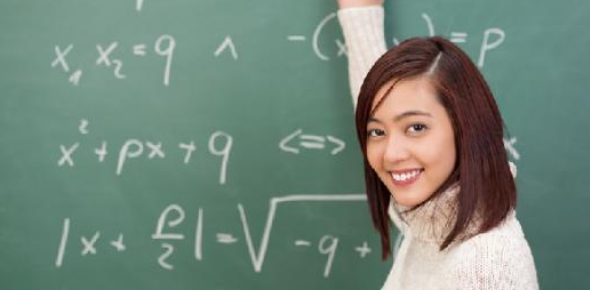# Grade 8 Mathematics Final Exam Practice Test I

30 Questions | Attempts: 7014SettingsWelcome to the Grade 8 Mathematics Final Exam Practice Test I. It's a medium level quiz for all the 8th graders out there who have their finals coming up and thus require practice sessions. With over 30 basic to medium level questions of maths, you can have a quality practice over the subject.

• 1.
MATH EXAM SECTION 1: MULTIPLE CHOICE Chapter 1 Representing Data Which of the following is not a type of graph?
• A.

Circle graph

• B.

Bar graph

• C.

Line graph

• D.

Square graph

• E.

Pictograph

• 2.
A music store was selling a special on a one hit single album, the manager made a graph on how much were sold during the 4 year period. How is the graph he made misleading?
• A.

• B.

Bars are to close

• C.

• D.

All of the above

• 3.
What is the BEST reason why a line graph is used to measure
• A.

Amount

• B.

Time

• C.

Large amounts

• D.

Percentage

• 4.
What is the BEST reason why a circle graph is used for?
• A.

Percent

• B.

Amount

• C.

Time

• D.

Large amounts

• 5.
What is the BEST reason why a pictograph is used to measure?
• A.

Amounts

• B.

Time

• C.

Percents

• D.

Large amounts

• 6.
SECTION II: SHORT ANSWER    A ____________ graph is used to measure certain data of distant amounts.
• 7.
Chapter 2 Rates, Ratios, and Proportions At a supermarket, there is a sale of a 4L jug of juice for \$3.99, a 2.5L bottle of Pop for \$2.49, and a 900mL bottle of soda for \$1.99. Which of the following is the best buy? (hint; convert L to mL)
• A.

Bottle of Pop

• B.

Bottle of Soda

• C.

Jug of Juice

• 8.
Three different kinds of pizzas have slices represented as the ratio: 3:6:12 Which of the following is an equal proportion?
• A.

6:36:144

• B.

12:24:72

• C.

450:900:1800

• D.

15:27:75

• 9.
To measure the equality between a rate and a ratio is called a (n) __________________
• 10.
Chapter 3 Pythagorean Relationship What is the following square root of x if x= 506
• A.

50.6

• B.

22.2

• C.

24.2

• D.

22.4

• 11.
Determine the possible number (2) on the scale between 1 and 2
• A.

2

• B.

1.4

• C.

4

• D.

None of the above

• 12.
Determine side "b" is a= 7cm, and c= 22cm of the right triangle:
• A.

435cm

• B.

43.5cm

• C.

20.8cm

• D.

22.5cm

• 13.
The Pythagorean theorem is _________________________ which is the formula used to measure the hypotenuse.
• 14.
Chapter 4 Percents What does the following diagram represent?
• A.

1013%

• B.

1.13%

• C.

113%

• D.

213%

• 15.
Which fraction is NOT equal to 20%?
• A.

2000/10000

• B.

800/2200

• C.

40,000/200,000

• D.

20/100

• 16.
A sale is going on at a sports store. A jersey is on sale for 20% off its original price of \$250.00, plus GST of 5% what is the total cost of the jersey?
• A.

\$210.00

• B.

\$185.00

• C.

\$200.00

• D.

\$168.00

• 17.
To find a ____________ , you times the decimal by 100
• 18.
Chapter 5 Surface AreaWhat object does this net form?
• A.

Rectangular prism

• B.

Triangular prism

• C.

Cylinder

• D.

Cube

• 19.
The formula to find the surface area of a ___________ prism is BxH/2
• 20.
• A.

5/6

• B.

20/24

• C.

0.83

• D.

10/12

• 21.
Determine the quotient of 3/4 divided by 6/5 in loswet terms
• A.

5/8

• B.

15/24

• C.

5/6

• D.

12/24

• 22.
Determine 5/6 x 6
• A.

10/72

• B.

6/30

• C.

5/35

• D.

None of the above

• 23.
The ___________ is the answer to a division question in fractions
• 24.
Chapter 7 VolumeWhat is the formula for determining volume of a prism?
• A.

Lxwxh

• B.

Volume is used on T.V!

• C.

BxH/2

• D.

BxH

• 25.
What is the formula for determining the volume of a cylinder?
• A.

Lxwxh

• B.

Bxh

• C.

Pi x r2 x h

• D.

2 x pi x r2

## Related TopicsBack to top
×

Wait!
Here's an interesting quiz for you.